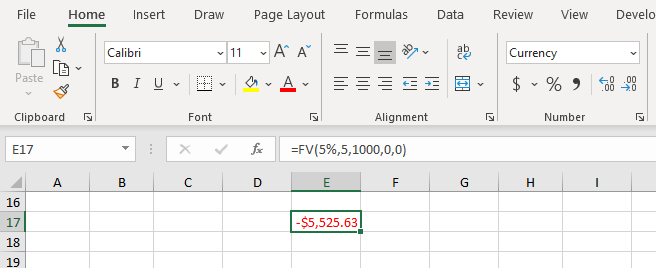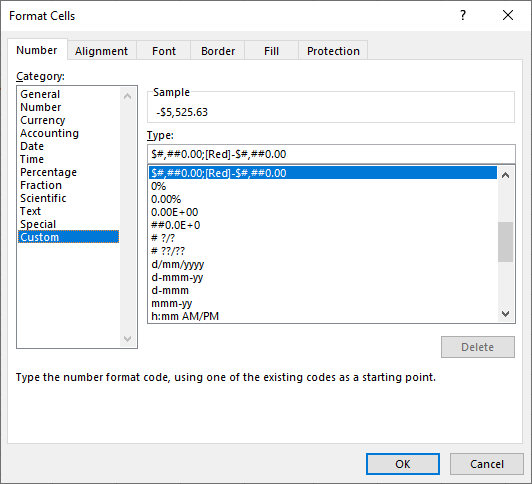# How to format the return value of a UDF

## 0. Apply format to custom function return value

1. An example of the Excel FV function with formatted return value
2. VBA code for UDF version
1. Annuity formula
2. Cash flow loop implementation
3. Format return value: VBA.Format, and Font.Color

## 1. WS functions with formatted return values

Excel functions from the Financial Category return values with a currency format applied. Examples include FV, NPV, PV, and PMT. An example of FV, the future value of an annuity is shown in figure 1.Fig 1: WS function - FV of ordinary annuity - with Currency format applied to return valueFig 2: Currency format - is equivalent to Custom format $#,##0.00;[Red]-$#,##0.00

## 2. VBA code

### 2.1 Currency format

Using the VBA macro recorder, the code to format Currency $1,234.45; -$1,234.45 is:

Selection.NumberFormat = "$#,##0.00_);[Red]($#,##0.00)"


### 2.2 Formula for annuity

The formula for the future value ($FV$) of an ordinary annuity is the sum of compounded future cash flows:

$$\begin{equation} FV = \sum\limits_{j=1}^{n} A \times (1 + i) ^ {n - j} = A \sum\limits_{j=1}^{n} (1 + i) ^ {n - j} \end{equation}$$

where:

• $A$ is the amount of the regular cash flow (payment)
• $i$ is the periodic interest rate
• $n$ is the number of regular cash flows (payments)

Equation 1 can be interpreted as the sum of a finite series, or equivalently, the partial sum of an infinite series. The sum $S_n$ is:

$$\begin{equation} S_n = a \left( \frac{(1-r)^n }{1-r} \right) \end{equation}$$ Substituting $(1 + i)$ for the common ratio $r$, and rearranging gives the familiar formula for the future value of an ordinary annuity:

$$\begin{equation} FV = A \times \left[ \frac{(1+i)^{n} - 1 }{i} \right ] \end{equation}$$

The syntax of the FV function is:

1. FV(rate,nper,pmt,[pv],[type])

With equation 3, the relationship to the FV function is: $\color{blue}{rate} = i$, $\color{blue}{nper} = n$, and $\color{blue}{pmt} = A$

### 2.3 The xlfFV UDF

The annuity formula, equation 3 is implemented in code 1.

Code 1: VBA code for equation 3
Function xlfFV(rate As Double, nper As Double, pmt As Double) As String
Dim tmp As Double
tmp = pmt * ((1 + rate) ^ nper - 1) / rate  ' equation 3
fssFV_2 = Format(-tmp, "Currency")
If tmp > 0 Then Selection.Font.Color = vbRed Else Selection.Font.Color = vbBlack
End Function


Currency format (the second part of the VBA Format function), code 1 line 8, and Color, code 1 line 9 are an approximation to the NumberFormat property.

The summation sequence, equation 1, is coded with a For...Next loop in code 2

Code 2: VBA code for equation 1 using a For...Next loop
Function fssFV_FNloop(rate As Double, nper As Double, pmt As Double) As String
' Returns the future value of an ordinary annuity
Dim tmp As Double
Dim j As Integer

For j = 1 To nper
tmp = tmp + pmt * (1 + rate) ^ (nper - j)     ' equation 1
Next j

fssFV_FNloop = Format(-tmp, "Currency")
If tmp > 0 Then Selection.Font.Color = vbRed Else Selection.Font.Color = vbBlack
End Function


• Development platform: Office 365 ProPlus Excel 64 bit.
• Published: 10 May 2020
• Revised: Wednesday 13th of May 2020 - 09:49 PM, Pacific Time (PT)